# Russo-Tartaglia-Sciortino model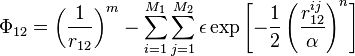$\Phi_{12} = \left(\frac{1}{r_{12}}\right)^{m} - \sum_{i=1}^{M_1}\sum_{j=1}^{M_2} \epsilon \exp \left[ -\frac{1}{2}\left( \frac{r_{12}^{ij}}{\alpha}\right)^n \right]$
where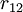$r_{12}$ is the distance between the centre of mass of particles 1 and 2,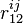$r^{ij}_{12}$ is the distance between patches$i$ and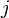$j$ on different particles, and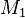$M_1$ and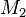$M_2$ are the number of patches of particles 1 and 2 respectively.